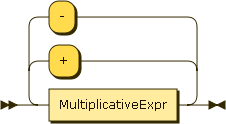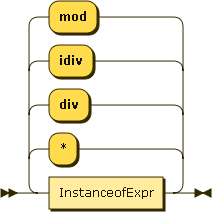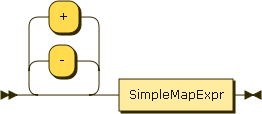### ArithmeticsFigure 31. MultiplicativeExprFigure 32. UnaryExprJSONiq supports the basic four operations, integer division and modulo.

Multiplicative operations have precedence over additive operations. Parentheses can override it.

Example 46. Basic arithmetic operations with precedence override

```1 * ( 2 + 3 ) + 7 idiv 2 - (-8) mod 2
```

Result (run with Zorba): 8

Dates, times and durations are also supported in a natural way.

Example 47. Using basic operations with dates.

```date("2013-05-01") - date("2013-04-02")
```

Result (run with Zorba): P29D

If any of the operands is a sequence of more than one item, an error is raised.

Example 48. Sequence of more than one number in an addition

```(1, 2) + 3
```

Result (run with Zorba): An error was raised: sequence of more than one item can not be promoted to parameter type xs:anyAtomicType? of function add()

If any of the operands is not a number, a date, a time or a duration, an error is raised.

Example 49. Null in an addition

```1 + null
```

Result (run with Zorba): An error was raised: arithmetic operation not defined between types "xs:integer" and "js:null"

If one of the operands evaluates to the empty sequence, then the operation results in the empty sequence.

Do not worry if the two operands do not have the same number type, JSONiq will do the adequate conversions.

Example 50. Basic arithmetic operations with an empty sequence

```() + 2
```

Result (run with Zorba):